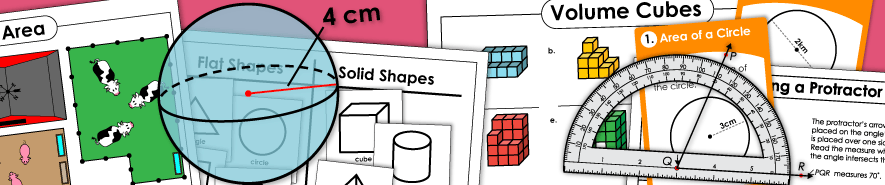# Geometry Worksheets - IndexAngles (Acute, Right, Obtuse)

Identify, classify, and draw acute, obtuse, and right angles.

Angles (Measuring)

Worksheets with practice problems for measuring angles with protractors.

Angles (Supplementary, Complementary, Vertical)

On this page, you'll find several worksheets for calculating the measurements of supplementary, complementary, and vertical angles.

Area Worksheets

Determine the area, or amount of space taken up, by the geometric shapes.

Area of Triangles

Use the formula a = 1/2 x (b x h) to calculate the area of triangles.

Flips, Slides, and Turns Worksheets

Practice sheets for shape movements. Examine the shapes to tell which were flipped, slid, or turned.

Ordered Pair Worksheets

Printable worksheets for plotting and finding ordered pairs on a coordinate plane.

Perimeter Worksheets

Add the sides to find the perimeters of the shapes.

Points, Lines, Segments, Rays

Learn about lines, line segments, rays, points, parallel lines, intersecting lines, and perpendicular lines.

Polygon Worksheets

Shapes for flat figures like rectangles, squares, triangles, hexagons, and parallelograms.

Similar and Congruent Shapes Worksheets

Worksheets for learning about translation, rotation, and reflection of shapes.

Solid Shapes Worksheets

Printable materials for teaching solid (3D) shapes like spheres, rectangular prisms, pyramids, cubes, and cones.

Solid Shapes Worksheets (Very Basic)

This page has very basic printables on identifying solid shapes. Includes cubes, cones, rectangular prisms, and spheres. Recommended for grades Pre-K through 1st.

Surface Area Worksheets

Determine the surface area of the cuboids (rectangular prisms) and other shapes.

Symmetry Worksheets

Tessellation Worksheets

Learn about geometric tessellations, or shapes that fit into each other to make a pattern.

Translation, Reflection, and Rotation Worksheets

Worksheets for learning about translation, rotation, and reflection of shapes.

Triangle Types

Classify triangles by sides (equilateral, scalene, and isosceles), and by angle (obtuse, acute, and right).

Volume Worksheets

Worksheets for calculating the volume of solids. Includes "counting cube" worksheets, as well as activities on using formulas to calculate the volumes of rectangular prisms, cones, pyramids, and spheres.